### Home > A2C > Chapter 4 > Lesson 4.2.3 > Problem4-105

4-105.
1. Think of absolute value as a statement about distance as you answer the questions below. What values of x in parts (a) through (d) make each equation true? Homework Help ✎

1. |x − 7| = 50

2. |x + 7| = 50

3. |10 − x| = 12

4. |2x + 1| = −3

5. What mathematical operation is best used for finding the distance between two numbers? In other words, if you wanted to know the distance from 42 to 117, what arithmetic expression would represent that distance?

6. Suppose you wanted to write an equation to represent the statement, “The distance between a number and 47 is 21.” You would not know whether to write x − 47 = 21 or 47 − x = 21. Absolute value equations can allow you to write a correct expression without knowing which value is larger. Write two absolute value equations that mean “the distance between a number and 47 is 21.”

7. Write and solve an absolute value equation that says each of the following:

1. The distance between x and 4 is 12.”

2. The distance between x and −9 is 15.”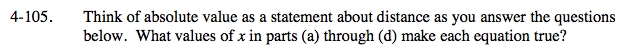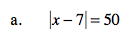What numbers are a distance of 50 from the number seven?

x = 57, −43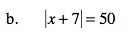See part (a).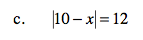See part (a).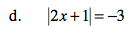See part (a).

Remember absolute values cannot be negative.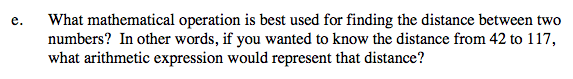Subtraction, 117 − 42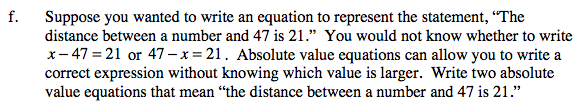|x − 47| = 21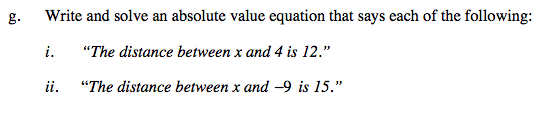See part (f).

|4 − x| = 12

|−9 − x| = 15